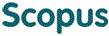### BROWSE

0 228

Cited 0 times in전상환자의 전혈수혈량 분석 및 소요량 추계 : 파월00부대를 중심으로

Other Titles
(An) estimation of whole blood volume needed for the war wounded based on the vietnam war
Authors
전기철
Issue Date
1982
Description
보건학과/석사
Abstract
[한글] 派越韓國軍○○部隊員 中 本國으로 後送되어 治療받은 788名의 醫務記錄에서 輸血量을 中心으로 資料를 募集, 分析하고 이를 基礎로 하여 全血所要量을 推計하였고 다음과 같은 決論을 얻었다. 1. 後送治療한 負傷者의 輸血量은 1人當 平均 5.6파인트였다. 2. 全體 後送治療者의 35.8%는 전혀 輸血을 받지 않은 것으로 나타났다. 3. 첫 응급처치를 받은 곳까지 後送하는데 所要된 時間이 짧을수록 投與된 輸血量은 增加하였다. 4. 總 輸血量의 59.4%는 負傷當日에 使用되었다. 5. 1人當 平均手術回數는 2.5回였다. 6. 1人當 平均手術時間은 205.3分이었다. 7. 總平均在院期間은 1人當 199.6日이었다. 8. 全血所要量의 推計公式 WBi = Ki[{0.8670Pg + 1.8188(1-Pg)}NWe + 0.5825 NEe]을 개발하였다. 단, WBi : 부상 I일의 필요 수혈량 Ki : I일의 수정계수 Pg : 부상원인 병기중 총상이 차지하는 율 NWe : 예상부상자 수 NEe : 예상사망자 수 Bi∈(B^^1, B^^3, B^^5, B^^7, B^^total) Ki∈(B^^1, K^^3, K^^5, K^^7, K^^total) K^^1 = 0.594 K^^3 = 0.626 K^^5 = 0.656 K^^7 = 0.668 K^^total = 1.000 * 표준오차 = 0.5507 ** 표준오차 = 0.5517
[영문] This study was carried out initially with the analysis of the blood volume used for the transfusion for the was wounded Korean Soldiers in Vietnam using the medical records of the patients transferred and treated in Korean Navy hospital, An equation was also developed for the estimation of blood volume which would likely to be needed in the future on the basis of analysis of this study. Followings are the brief summary of the findings. 1. The total average volume of blood transfusion was estimated to be 5.6 pints per wounded patient in general and 11.2 pints of whole blood were estimated to be required for the abdomen - wounded patient and 6.1 pints for the thorax - wounded patients. 2. 35.8 percent of total wounded patients received no transfusion. 3. It appeared that the time required for the referral increased the required volume of blood transfusion decreased. 4. Among the total volume of blood transfused 59.4% of total transfusion was made on the very day of the wound. 5. Average number of operation was 2.5 per patient. 6. Average minutes required for surgery appeared to be 205.3 minutes per patient. 7. Total length of stay was 199.6 days per patient in average. 8. An equation for the estimation of future whole blood requirement was developed. WBi = Ki[{0.8670Pg + 1.8188(1-Pg)}NWe + 0.5825 NEe] Where WBi = Whole blood would be needed Ki = Constant of ith day of wound Pg = Proportion of Gun-shot wounded among all wounded NWe = Number of wounded expecting NEe = Number of Exipire expecting Bi∈(B^^1, B^^3, B^^5, B^^7, B^^total) Ki∈(B^^1, K^^3, K^^5, K^^7, K^^total) K^^1 = 0.594 K^^3 = 0.626 K^^5 = 0.656 K^^7 = 0.668 K^^total = 1.000 * Standard error = 0.5507 ** Standard error = 0.5517
Appears in Collections:
4. Graduate School of Public Health (보건대학원) > Others (기타) > 2. Thesis
URI
https://ir.ymlib.yonsei.ac.kr/handle/22282913/116855
사서에게 알리기